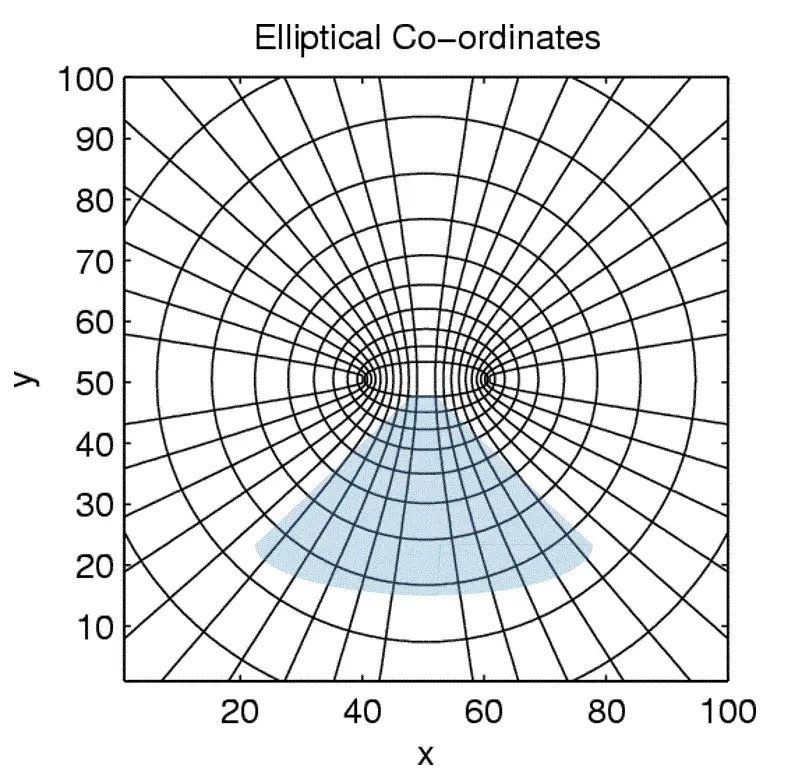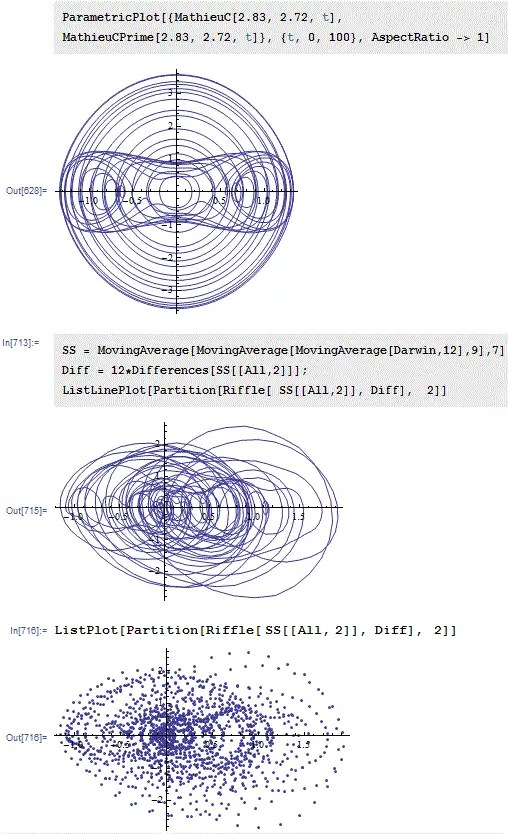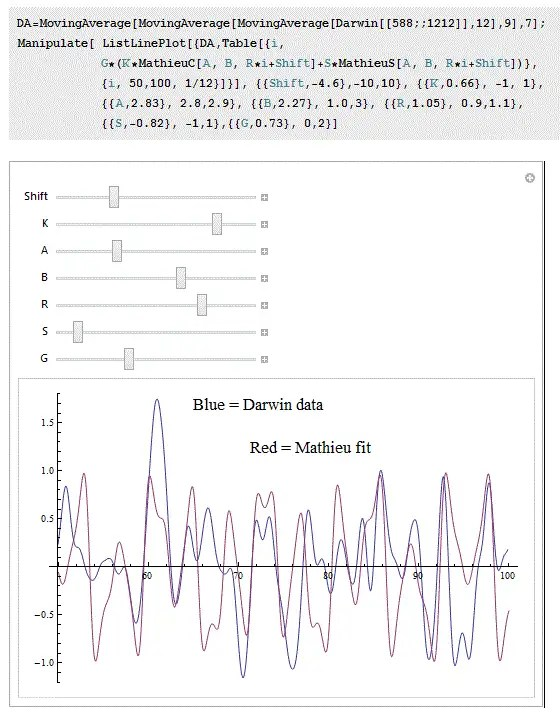The Southern Oscillation Index Model

(Note dated 4/18/2019 — an update from the future. This was the first in a long series of posts trying to understand ENSO, which finally culminated in a publication described here on Book Info
(also see later posts on this blog here))

[mathjax]A simple model of the Southern Oscillation Index (SOI) does not exist. I find it important to understand the origin of the SOI fluctuations, not only because it is an interesting scientific problem but for its potential predictive value — in particular,  I could use a model to extrapolate the SOI factor needed by the CSALT model to make global surface temperature projections.  This has implications not only for long-term climate projections but for medium-term seasonal weather predictions, particularly in predicting the next El Nino.

The current thinking is that the index that characterizes the presence of El Nino and La Nina conditions (also known as ENSO)  is unpredictable enough to make any prediction beyond a  year or two pointless. That makes it a challenging problem, to say the least.

So although the SOI is defined as oscillatory (thus the name), these oscillations are not the typically sinusoidal, perfectly periodic waveforms that we are used to dealing with, but consist of uneven, sporadic pulses that remain virtually impossible to deconstruct. Yet, the problem may not be as intractable as we are lead to believe. The key to understanding the SOI is to decode the characteristics of the waveform itself shown below in Figure 1.Fig 1 : The SOI is defined as the atmospheric pressure difference between Darwin, Australia and Tahiti in the south Pacific. Given that we have measurements that span over 130+ years, there may be a possibility that we can crack the code and decipher the fluctuating waveform. In the figure, the sloped dotted line gives us a clue to their nature.

The waveform is periodic alright but this is the periodicity that lies in the strange mathematical world of crystal lattices and warped coordinate systems.  Follow the math on the next page and the mystery is revealed.

The first piece of evidence that suggests a promising derivation is shown by the slanted dotted line in Figure 1.  Although only faintly visible, an envelope of a higher order period is discernable within the fluctuating waveform.  This is very reminiscent of a Bloch wave, which occurs as a solution to a particle residing within potential energy wells modulated by a periodic lattice.Figure 2: A schematic of a typical Bloch wave in one dimension. Note that the waveform created by a periodic lattice of energy wells is modulated over a longer period.

What happens is that the waveform of an electron propagating within a crystal lattice will interfere with the waveform of the lattice itself to define the band-gap structure of the electronic material. Since crystal lattices are my area of academic expertise, I know enough that solving Schrodinger’s equation doesn’t apply to the vast expanse of the Pacific Ocean. But we do know that boundary conditions and the shape of the ocean itself are enough to set up the equivalent of standing waves and propagating waves of complicated structure within the ocean medium.  The motivating factor is that once one has seen how much work goes into calculating the strangely periodic band-structure of Silicon and Gallium Arsenide semiconductors, the SOI looks a bit like child’s play in comparison (only half-joking).

The usual math formulation applied to these kinds of problems is referred to as the wave equation. This is a partial differential equation that includes spatial and temporal dimensions.

$${partial ^{2}z over partial t^{2}}=c^{2}nabla ^{2}z$$

in two dimensions, this becomes

$${1 over c^2}{partial ^{2}z over partial t^{2}}= {partial ^{2}z over partial x^{2}} + {partial ^{2}z over partial y^{2}}$$

Solutions to this class of partial differential equation are critically dependent on the boundary conditions of the problem.  The other important aspect is to be able to separate the dimensions of variability in the problem domain. This means that if we can separate the temporal from the spatial, and then separate the two spatial dimensions (x and y), we are off and running with a potential solution that we can check against the data.

The observation that I turn into a premise relies on the similarity of the Pacific Ocean basin to a portion of an elliptical coordinate system, as shown in Figure 3 below.Fig. 3 : A solution context that resembles the irregular structure of the Pacific Ocean basin is defined by elliptical coordinates. The X-axis (East-West) can be described via a rotational measure, while the Y-axis (North-South) is a hyperbolic measure. The blue shaded area describes the mapping with the ENSO creating waves in the East-West dimension.

Such an elliptical coordinate system is used to solve problems that do not exhibit rectangular, cylindical, or perfectly spherical symmetry.  A decomposition of the wave equation onto an elliptical coordinate system is aided by the application of the foreboding world of Mathieu functions.  Keep in mind that since Arfken  has warned us that “Mathieu functions to be among the most difficult special functions used in physics”, we proceed with caution and trepidation, but ultimately realize that you often have to do what you have to do. And so this is where we start.

Wave Equation in Elliptical Coordinates

I will get into more detail in a future post but with the aid of the excellent resource of Arfken’s “Mathematical methods for physicists”  and the Mathematica web pages on Mathieu Functions, we can skip many of the derivation steps to arrive at a suitable formulation that we can evaluate.  As a few starting definitions, we introduce a variable relating space to time via a propagating or traveling wave:

$$eta = x – vt$$

and to represent a forcing strength a linear dispersion relating the wave vector to frequency:

$$q = frac{c^2 {omega}^2}{4v^2}$$

These wave-based identities are applied to transform the partial differential wave equation into an ordinary differential equation (ODE), which is more easy to solve — if you don’t mind working with hideous transcendental functions not available within an Excel spreadsheet.

The following ODE is known as the Mathieu equation.  Although relatively innocuous-looking, the nonlinear recursive interaction of the Φ = Phi term (which represents the observable measure) and the cosine factor makes it impossible to solve in terms of other trigonometric identities.

$$frac{d^2Phi}{deta^2}+[a-2qcos(2eta)]Phi=0$$

Fortunately, we have an out available and we can find analytical solutions if we are not afraid to apply the special-case functions known by MathieuC and MathieuS.  These are the elliptical trigonometric analogues to the familiar Cosine and Sine function used to form the basis set of periodic solutions in a linear ODE. (Note that for a small enough q, we recover the perfect sinusoidal result).

As a kernel solution to the above Mathieu equation, we can theoretically assume a linear combination of the two basis Mathieu functions, where a is the characteristic value (a form of eigenvalue) and q is the parameter as described in the ODE. The constants c1 and c2 are determined by the initial conditions.

z(t) = c1 MathieuC(a, q, t) + c2 MathieuS(a, q, t)

The next step is to apply this result to a physical phenomenon; in this case that of the measurable SOI time series parameter. Since the SOI is formally defined as the atmospheric pressure difference between measurements made at Tahiti and Darwin, we begin with the historical data set from the Darwin location.

As an initial exploratory step, we create a parametric plot relating the SOI value against its first derivative. A purely sinusoidal waveform will appear as a circle.  However, a Mathieu function will appear as an elliptically-convoluted orbit as shown in Figure 4. The Darwin data is shown in the two lower panels.Fig 4: A time series showing characteristics of a Mathieu function will feature specific Lissajous patterns in parametric plots of the value against its first derivative. The top plot is a perfect realization and the bottom two are line and point plots of the Darwin time series, which bring out the “head peering through a binocular” effect.

The agreement is reassuring so the next step is to linearize the data according to the ODE.  Rearranging the ODE, we want to see if we can extract the characteristic value, strength parameter, and the basic frequency:

$$frac{d^2Phi}{deta^2} = – [a-2qcos(2eta)]Phi$$

By numerically calculating the second derivative from the Darwin time-series data as a LHS value and then minimizing the error with respect to the RHS value, we can estimate these values.Fig. 5 : Plotting the minimal error fit between LHS and RHS for values of a, q, and base frequency.

The R^2 values are not very good but we are trying to find any kind of agreement so that we will live with these values for the moment.  The following values come from applying a solver:

• a = 2.83
• q = 2.72
• T = 6.30 years

Note that T is close to the third harmonic of the diurnal Kola cycle or Lunar standstill used in the CSALT model.

So the last step is the most amazing of all.  We take the values as estimated from the solver and use these to parameterize the Mathieu functions, and then compare it to an interval of the Darwin data from the yearsFig. 6 : Fit of the Mathieu SOI Model in RED to Darwin data in BLUE. This is a 50 year interval from 1930 to 1980.

This looks reasonable, so what happens if we project the Mathieu SOI model to 2080?Fig. 7 : Projecting the Mathieu SOI model in RED fit to the 1930-1980 training interval data in BLUE to the present day and beyond to 2080.

The model does not capture the extended El Nino conditions of the 1980-2000 interval and it actually inverts the early 1990’s high pressure Darwin anomaly.  But notice that it does have a long term repeat interval of 40 years as indicated by the Bloch-wave-like envelope.  This 40-year number has long been identified as a significant climate periodicity. The elliptical-premise SOI Model clearly has potential.

Summary

The SOI is defined as the atmospheric pressure difference between Darwin, Australia and Tahiti in the south Pacific. Atmospheric pressure has the strong physical property that it must revert to a long-term mean of the earth’s sea-level pressure. This means that an excursion beyond the mean for any length of time can not be sustained and that it eventually has to settle back to zero and then oscillate in the other direction.  Since the SOI is a significant factor in the CSALT model of global temperature, and that it goes a long way to explaining the pause in current temperatures, any model of SOI is welcome as a tool to making climate projections.  Other researchers have modeled the SOI as red noise , while there is a strong contingent that calls the chaos card and asserts that models are impossible due to irreducible uncertainty within the natural environment.

One thing left to do is fit to the actual SOI — as we apply Mathieu models with the correct aligning phase shifts for each location, and then difference the two time-series.  This can be compared to the actual data. More on that later.

In summary, given that we have measurements in SOI that span over 130+ years, there is now a strong possibility that we can crack the code and decipher the fluctuating waveform. Mathieu functions and their brethren have long been used to model the complex solid-state physics behind all modern-day electronics.  And so it appears that a similar Mathieu-based SOI Model gives us a clue to the mathematics behind the unpredictable nature of the El Nino. It’s another of those predictably unpredictable behaviors that seem to proliferate in nature, but this one may be cracked completely.

References

1. Arfken, George Brown, Hans-Jurgen Weber, and Lawrence Ruby. Mathematical methods for physicists. Vol. 6. New York: Academic press, 1985. See http://booksite.elsevier.com/9780123846549/index.php for a freely available supplement describing Mathieu function.
2. Privalsky, Victor, and Sergey Muzylev. “An Experimental Stochastic Model of the El Niño–Southern Oscillation System at Climatic Time Scales.”,  Universal Journal of Geoscience 1(1): 28-36, 2013, http://www.hrpub.org, DOI:10.13189/ujg.2013.010104(2013).

23 thoughts on “The Southern Oscillation Index Model”

1.Dennis Coyne

Keep in mind that the Tahiti Data before1930 or so is not very good.

“The SOI values prior to 1935 should be used with caution. There are questions regarding the consistency and quality of the Tahiti pressure values prior to 1935. ”

Like

2.admin

DC, Yes, thanks, I remembered that and that’s why I stayed with the Darwin data.

Like

3.admin

A very short section (25ms) of an inharmonic waveform (cymbal).

Like

•admin

Thanks David, I have seen that data before and actually got sick of seeing it, as the irritating denialist Chief Hydrologist would whip that out quite often without presenting any context. . It is possible that different orbital forcings will change the frequency.

Like

4.Dennis Coyne

Hi Paul,

A quick question. Is your plan to do the same analysis on the Tahiti Data, maybe from 1935 to 1985 and then create simulated Tahiti data (based on your Tahiti model)from 1880 to 1935, and then you could create a simulated SOI from 1880 to 1935 where simulated SOI is Darwin data minus simulated Tahiti data up to 1935? Then you could splice together the whole series from 1880 to 2013 and see how the CSalt comes out. Aerosols are impossible to predict, so you could leave these out of your model (CSLT). Finally you could use a several periodic functions to replace LOD (60 year) and T(11 or 22 year or both) so that you would only have CS plus some periodic functions. Then perform the regression on just these(C and S) parameters along with chosen periodic functions over the period from 1880 to 2013 to get coefficients that match the Temperature data. Then an estimate of future carbon dioxide levels in the atmosphere could be created to do a forward projection of Temperature levels (based on fast feedbacks only). I actually have such a projection of carbon dioxide levels based on your shock model which I applied to coal, oil and natural gas and likely ultimately recoverable reserves. If your interested let me know.

Like

•admin

DC,
That’s about right on with respect to my plan. Model the Tahiti data and go from there. As an interim analysis, I am looking at the frequency spectra and making sure that also makes sense.

It is possible that the LOD may be an orthogonal component to the SOI oscillations, which fluctuates north-south instead of the east-west SOI. The idea behind the elliptical decomposition was to isolate the factors and it may be that LOD is the other ellipse axis in the Mathieu formulation. That function does not oscillate as much and is apparently even more obscure.

I am not worried about the projected CO2 levels as much, as that would be a parametric input anyways.

Like

5.Tom Gebhart

Hey Paul,

Couple questions:

Is there any other evidence that the Southern Oscillation Index can be modeled by jumping off from a Bloch wave? I would like to know how you came to your conclusion to base the model off of this physical feature.

Also, what is the significance of the T parameter being close to the diurnal Kola Cycle used in the CSALT model? I’ve looked over the link but wasn’t able to make the connection.

Like

•admin

Tom, The Bloch wave is essentially an analogy based on the similarity of the functional form. The Mathieu equation is a specific variation of the Hill equation, which is applied to the Schrodinger equation in a periodic crystal lattice. That gives rise to Bloch waves. I am not sure if any geophysicists have made the connection, but they also probably haven’t studied solid-state physics either.

The reason that I apply it to ocean basins is two-fold. One, the elliptical symmetry of the area and depth contours force a cos/cosh curvature to the two-dimensional wave equation. See the first reference for how this is derived. Second, it is clear that a yearly and multiples of a year periodic impulse, partly based on tidal forcing, is applied to the system which can reinforce or resonate with the Mathieu equation.

As to the 6.3 year cycle, I have gone into a bit more detail in the next post
http://contextearth.com/2014/02/21/soim-and-the-paul-trap/
The fundamental frequency of a Mathiue equation with a applied period of 6.3 and a-value of 2.83 is 6.3/sqrt(2.83)=3.75 years or about 45 months. This value shows up in Fourier series analyses as well:
http://www.mathworks.com/help/curvefit/fourier-analysis-of-enso-data.html

Hopefully the next post can clear some of these questions up, and probably raise other ones.

Like

6. Pingback: ENSO redux | context/Earth

7.Joline Strauch

Like

8.Paulo Lorenzo
9. Pingback: Confirmation Bias | context/Earth

10. Pingback: AMO | context/Earth

11. Pingback: PDO | context/Earth

12. Pingback: PDO | context/Earth